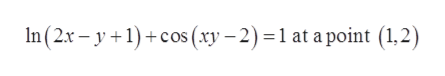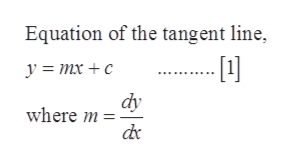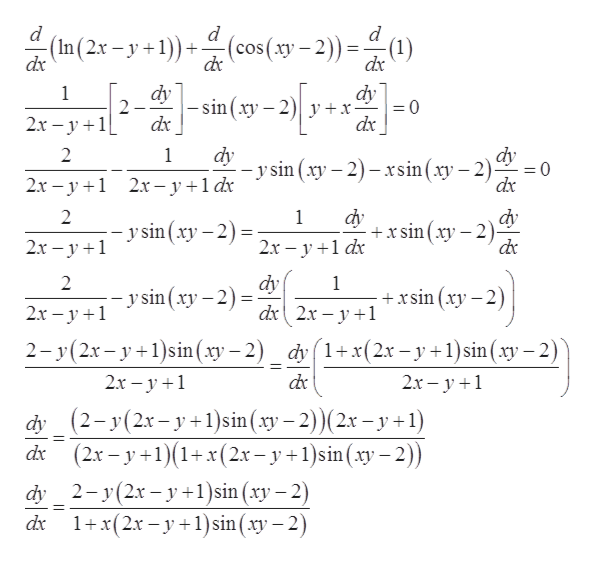# Find an equation of the tangent line to ln(2x-y+1) + cos(xy-2)=1 at the point (1,2)

Question
38 views

Find an equation of the tangent line to ln(2x-y+1) + cos(xy-2)=1 at the point (1,2)

check_circle

Step 1

Given:help_outlineImage TranscriptioncloseIn (2x – y +1) +cos (xy – 2) =1 at a point (1,2) fullscreen
Step 2

Concept used:help_outlineImage TranscriptioncloseEquation of the tangent line, --  у %3 тх + с dy where m = de fullscreen
Step 3

Find dy/dx from the given equation by differen...help_outlineImage Transcriptionclose(In (2x - y +1))+ (cos(xy – 2)) =(1) dx de dx dy dy -sin(xy – 2)| y +x- dx 2.x – y +1L dx dy - y sin (xy – 2) – xsin(xy – 2) dx dy %3D 2х - у +1 2х-у+1dх dy dy +x sin (xy – 2)- de -- y sin (xy – 2) = 2.x – y +1 2х — у +1 dx dy -y sin (xy – 2) = +xsin (xy – 2) dx 2x – y +1 2x – y +1 dy 1+x(2x– y +1)sin (xy – 2) 2- y(2x– y +1)sin(xy – 2) 2х - у +1 de 2х - у +1 dy (2-у(2х-у +1) sin (ху — 2)) ( 2х - у +1) (2x – y +1)(1+x(2.x – y +1)sin(xy – 2)) dx 2-у (2х — у+1)sin (ху — 2) 1+x(2x – y +1)sin(xy – 2) dy dx fullscreen

### Want to see the full answer?

See Solution

#### Want to see this answer and more?

Solutions are written by subject experts who are available 24/7. Questions are typically answered within 1 hour.*

See Solution
*Response times may vary by subject and question.
Tagged in

### Derivative